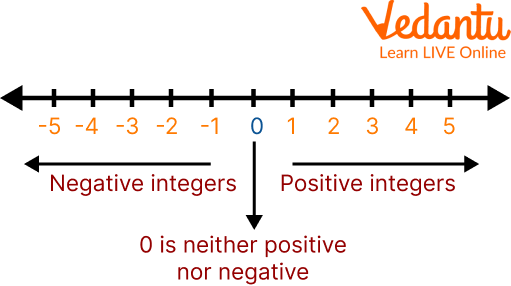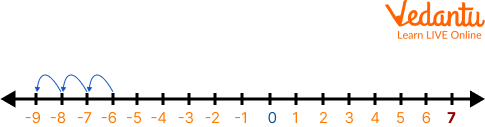Courses
Courses for Kids
Free study material
Free LIVE classes
More

# Addition of Numbers Using A Number LineLIVE
Join Vedantu’s FREE Mastercalss

## Introduction to Addition on Number Line

The addition on the number line helps us to visually perform the addition operation on small numbers. A number line is a visual representation of numbers on a straight line where the value of the numbers increases as we move from left to right. Arithmetic operations like addition, subtraction, multiplication, and division can be performed on a number line. In this article, you will learn about number lines and their concepts. How to add and subtract a number line will be explained in this article, and some solved examples and practice problems will also be shown for your help.Number Line

## Addition of Numbers Using A Number Line

Addition on number line is as simple as counting positive numbers by moving towards the right-hand side of a number line. It helps us to visually understand the addition operation using small numbers.

By utilising small numbers, the sum on the number line makes the addition operation easier to understand visually. Moving to the right makes it simple to count positive numbers.

• So to add the negative number, move to the left.

• To add the positive number, move to the right.## Addition of a Positive Number to a Positive Number

The addition of a positive number to a positive number means that both numbers will be positive. Here we will mainly look at adding a positive number to a positive number. Let’s understand it through an example:

Solution: Let us look into the steps below to understand addition on a number line.Step 1: Consider the first number (1) as the starting point on the number line. To add 1 + 2, mark 1 on the number line.

Step 2: Now, from the first number, jump by the number of units equivalent to the second number towards the right. This is because the values on a number increase as we move towards the right-hand side. In this case, we are adding 1 + 2. Therefore, we will move 2 steps to the right. This will bring us to number 3. So, 1 + 2 = 3

## Addition of a Negative Number to a Positive Number

Here we will mainly look at the addition of a negative number to a positive number:

To add a negative number to a positive number, we always move to the left on the number line. Let us take an example to understand this.

Ans: Here, we take 3 as the starting number and move 4 units towards the left on the number line, which gives the result as -1.Addition of a Positive Number to a Negative Number

## Addition of a Positive Number to a Negative Number

We always move to the right on the number line to add a positive number to a negative number. Let us take an example to understand this.

Example: Add -6 + (3) = -3

Ans: Here, we take -6 as the starting number and move 3 units towards the right on the number line, which gives the result as –3.Addition of a Positive Number to a Negative Number

## Addition of a Negative Number to a Negative Number

To add a negative number to a negative number, we always move to the left on the number line. Let us take an example to understand this.

Example: Add -6 + (-3) = -9

Ans: Here, we take -6 as the starting number and move 3 units towards the left on the number line which gives the result as –9.Addition of a Negative Number to a Negative Number

## Solved Examples

Here the addition of numbers using number line-related examples are described, which are as follows:

Q 1. Addition (-4) with 3.

Ans: In this case, we start with -4 and move 3 units to the right on the number line, yielding the result -1.

Use an addition of numbers using the number line method,Addition of -4 with 3 on Number Line

Q 2. Addition 4 with 3.

Ans: In this case, we start with 4 and move 3 units to the right on the number line, yielding the result 7.

Use an addition of numbers using the number line method,Addition of 4 with 3 on Number Line

## Practice Problems

Solve these additions by using the number line:

Q 1. \$4+(-5)=\$

Q 2. \$-2+2=\$

Q 3. \$-3+7=\$

Q 4. \$2+(-5)=\$

1. -1

2. 0

3. 4

4. -3

## Benefits of Using a Number Line

In this section, we will talk about the importance of using a number line in daily life. The benefits of using a number line are:

• It helps teach children the concept of place value by comparing digits and ordering them according to their value;

• It helps children count by arranging numbers from least to greatest;

• It can be used for mental math, such as adding and subtracting;

• It can help students understand how fractions work by representing them with parts of a whole.

## Summary

The number line is a simple and easy way to show the relationship between numbers. It is used in mathematics, science, and finance. In daily life, number lines teach children to read numbers. A number line is a mathematical tool that can help people understand how numbers work. A number line is a straight line in mathematics with numbers spread at equal intervals or segments along its length. The article on number lines explains how a number line can be stretched indefinitely in any direction and how it is typically depicted horizontally.

Last updated date: 23rd Sep 2023
Total views: 81.9k
Views today: 2.81k

## FAQs on Addition of Numbers Using A Number Line

1. What exactly is the number line rule?

Comparing numbers is made simpler by writing them on a number line. The numerals on the left of the number line are smaller than those on the right. You can perform addition, subtraction, and multiplication using a number line. To add, subtract, or multiply, we always advance to the right or left or by skipping a count.

2. What is the purpose of a number line?

A number line is a visual math tool, making it helpful to add and subtract numbers. It can assist parents and educators in helping kids learn to count and write numbers. It can also be used to develop comprehension of the concepts involved in addition, subtracting, multiplying, and dividing numbers.

3. Where do number lines appear in practice?

Number lines are a constant in our daily life. A road is a very long number line when we are driving. When measuring the length or capacity of a liquid measuring cup, we use number lines.